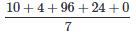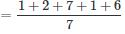Courses

# Test: Calendar 2

## 20 Questions MCQ Test UPSC Prelims Paper 2 CSAT - Quant, Verbal & Decision Making | Test: Calendar 2

Description
This mock test of Test: Calendar 2 for Quant helps you for every Quant entrance exam. This contains 20 Multiple Choice Questions for Quant Test: Calendar 2 (mcq) to study with solutions a complete question bank. The solved questions answers in this Test: Calendar 2 quiz give you a good mix of easy questions and tough questions. Quant students definitely take this Test: Calendar 2 exercise for a better result in the exam. You can find other Test: Calendar 2 extra questions, long questions & short questions for Quant on EduRev as well by searching above.
QUESTION: 1

### What was the day of the week on 10th March 1996?

Solution:

== 134/7
=1
= Sunday

QUESTION: 2

### What day of the week was 20th June 1837?

Solution:

20th June, 1837 means 1836 complete years + first 5 months of the year 1837 + 20 days of June
1600 years give no odd days
200 years give 3 odd days
36 years give (36 + 9 ) or 3 odd days
Thus 1836 years give 6 odd days
From 1st January to 20th June there are 3 odd days
Odd days: January 3, February 0, March 3, April 2, May 3, June 6 = 17
Thus the total number of odd days = 6 + 3 or 2 odd days
This means that the 20th of June fell on 2nd day commencing from Monday.
The required day was Tuesday.

QUESTION: 3

### How many days will there be from 26th January, 1996 to 15th May, 1996 (both days included)?

Solution:

Number of days from 26-Jan-1996 to 15-May-1996 (both days included)
= 6(Jan) + 29(Feb) + 31 (Mar) + 30(Apr)+ 15(May)
= 111

QUESTION: 4

Today is Thursday. What will be the day of the week after 94 days?

Solution:

94 days = (13 × 7) + 3 = 3 odd days.
The required day is 3 days beyond Thursday i.e., Sunday

QUESTION: 5

What was the day on 25th January, 1975?

Solution:

Counting the years 1600 + 300 + 74
In 1600 years, there are zero odd days
In 300 years, there is one odd day
In 74 years, there are 18 leap years and 56 normal years, so the odd days are:
18(2) + 56(1) = 36 + 56 = 92,
Which is 13 weeks and 1 odd day
In 25 days of January, 1975, there are 3 weeks and 4 odd days
Total odd days = 0 + 1 + 1 + 4
six odd days, so it was a Saturday.

QUESTION: 6

Arun went for a movie nine days ago. He goes to watch movies only on Thursdays. What day of the week is today?

Solution:

Clearly it can be understood from the question that 9 days ago was a Thursday
Number of odd days in 9 days = 2 (As 9 - 7 = 2, reduced perfect multiple of 7 from total days)
Hence today = (Thursday + 2 odd days) = Saturday

QUESTION: 7

On 2007, What was the date of last Saturday in May month?

Solution:

1 - May - 2007=17/7
=3
=Tuesday
= May 1st → Tuesday + 5 days = Saturday = 5th may
5th may + 7 days = Saturday = 12th may
12th may + 7 days = Saturday = 19th may
19th may + 7 days = Saturday = 26th may
= Answer = 26th may

QUESTION: 8

If the seventh day of a month is three days earlier than Friday, What day will it be on the nineteenth day of the month?

Solution:

Given that seventh day of a month is three days earlier than Friday
⇒ Seventh day is Tuesday
⇒ 14th is Tuesday
⇒ 19th is Sunday

QUESTION: 9

Today is 3rd November. The day of the week is Monday. This is a leap year. What will be the day of the week on this date after 3 years?

Solution:

This is a leap year. So, none of the next 3 years will be leap years. Each year will give one odd day so the day of the week will be 3 odd days beyond Monday i.e. it will be Thursday.

QUESTION: 10

If 1st October is Sunday, then 1st November will be

Solution:

Given that 1st October is Sunday
Number of days in October = 31
31 days = 3 odd days
(As we can reduce multiples of 7 from odd days which will not change anything)
Hence 1st November = (Sunday + 3 odd days) = Wednesday

QUESTION: 11

Find the number of odd days in 123 days

Solution:

Odd days ⇒ The number of days more than complete number of weeks in the given period are odd days.
123 = 7 × 19 + 4 ⇒ 4 odd days.

QUESTION: 12

Given that on 18th April 1603 is Thursday. What was the day on 18th April 2003?

Solution:

After every 400 years, the same day occurs. (Because a period of 400 years has 0 odd days)
Thus, 18th April 1603 is Thursday, After 400 years i.e., on 18th April 2003 has to be Thursday.

QUESTION: 13

Today is Friday, after 126 days, it will be:

Solution:

126/7
= 0
Each day of the week is repeated after 7 days.
So, after 126 days, it will be Friday.
After 126 days, it will be Friday

QUESTION: 14

If 25th of August in a year is Thursday, the number of Mondays in that month is

Solution:

Given that 25th August = Thursday
Hence 29th August = Monday
So 22nd,15th and 8th and 1st of August also will be Mondays
Number of Mondays in August = 5

QUESTION: 15

How many times does the 29th day of the month occur in 400 consecutive years?

Solution:

In 400 consecutive years there are 97 leap years. Hence, in 400 consecutive years February has the 29th day 97 times and the remaining eleven months have the 29th day 400 × 11 or 4400 times.
Thus the 29th day of the month occurs
= 4400 + 97
= 4497 times.

QUESTION: 16

Second Saturday and every Sunday is a holiday. How many working days will be there in a month of 30 days beginning on a Saturday?

Solution:

Mentioned month begins on a Saturday and has 30 days
Sundays = 2nd, 9th, 16th, 23rd, 30th
⇒ Total Sundays = 5
Every second Saturday is holiday.
1 second Saturday in every month
Total days in the month = 30
Total working days = 30 - (5 + 1) = 24

QUESTION: 17

December 9, 2001 is Sunday. What was the day on December 9, 1971?

Solution:

Total number of days = 30 × 365 + 8 days from leap years = 10958
Thus number of weeks = 1565
Hence December 9, 1971 must have been Tuesday.

QUESTION: 18

What day of the week was 1 January 1901

Solution:

1 Jan 1901 = (1900 years + 1st Jan 1901)
We know that number of odd days in 400 years = 0
Hence the number of odd days in 1600 years = 0 (Since 1600 is a perfect multiple of 400)
Number of odd days in the period 1601 - 1900
= Number of odd days in 300 years
= 5 x 3 = 15 = 1
(As we can reduce perfect multiples of 7 from odd days without affecting anything)
1st Jan 1901 = 1 odd day
Total number of odd days = (0 + 1 + 1) = 2
2 odd days = Tuesday
Hence 1 January 1901 is Tuesday.

QUESTION: 19

Today is 5th August. The day of the week is Wednesday. This is a leap year. What will be the day of the week on this date after 3 years?

Solution:

This is a leap year.
So, none of the next 3 years will be leap years.
Each ordinary year has one odd day, so there are 3 odd days in next 3 years.
So the day of the week will be 3 odd days beyond Wednesday i.e. it will be Saturday

QUESTION: 20

January 1, 2004 was a Thursday, what day of the week lies on January 1 2005.

Solution:

Given that January 1, 2004 was Thursday.
Odd days in 2004 = 2 (because 2004 is a leap year)
(Also note that we have taken the complete year 2004 because we need to find out the odd days from 01-Jan-2004 to 31-Dec-2004, that is the whole year 2004)
Hence January 1, 2005 = (Thursday + 2 odd days) = Saturday